# MCQs on Electric Circuits

##### Page 17 of 63. Go to page 1 2 3 4 5 6 7 8 9 10 11 12 13 14 15 16 17 18 19 20 21 22 23 24 25 26 27 28 29 30 31 32 33 34 35 36 37 38 39 40 41 42 43 44 45 46 47 48 49 50 51 52 53 54 55 56 57 58 59 60 61 62 63
01․ The voltage across R and L in a series RL circuit are found to be 200 V and 150 V respectively the rms value of the voltage across the series combination is ______ V.
360
250
200
450

Voltage across series combination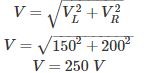02․ Three identical resistor are connected in star across a 3 phase 550 V supply dissipating a total power of 300 W the line current is ____________ A.
3.149
0.5
1
2.56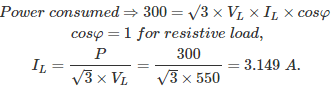03․ A motor having a power factor of 0.8 absorbs in active power of 1200 W the reactive power drawn from the supply is _________ VA.
130
900
250
400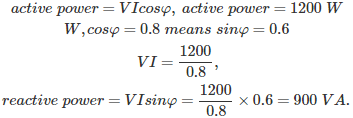04․ A coil of negligible resistance has an induction of 100 mH. The current passing through the coil changes from 2 A to 4 A at a uniform rate in 0.1 sec the voltage across the coil during this time would be __________ V.
2
8
36
50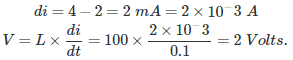05․ The instantaneous values of line current into a delta connected load in any two lines are + 2.5 amps and - 1.25 amps the current in the third line at the instant is ___________ A.
1
- 1.25
10
2.5

Since in a delta connected system at any instant the vector sum of current must be zero. ∴ +2.5 - 1.25 + I = 0, ∴ I = -1.25 amps.

06․ A current of 4 A flows in an ac circuit when 100 V dc is applied to it whereas it takes 250 v ac to produce the same current the power factor of the circuit is
0.4.
10.
1.
0.85.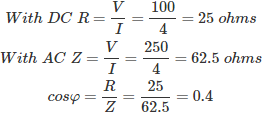07․ The strength of current in 1 H inductor changes at rate of 1 A / sec. Find the voltage across it?
2 V.
1 V.
0.5 V.
None of these.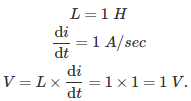08․ Constant K type HPF (high pass filter) having cut off frequency 12 KHz and nominal impedance Ro = 500 Ω Find shunt arm inductor L and series arm capacitance C for T and π section of HPF ?
L = 0.0132 mH, C = 0.0132 μF.
L = 3.316 mH, C = 0.0132 μF.
L = 3.316 mH, C = 132 μF.
None of these.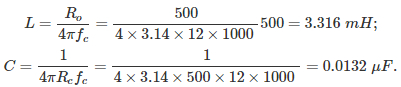09․ In foster 1 form 1st element is
capacitor.
inductor.
both.
none.

In foster 1 form 1st element Co (pole at Ω = 0) and last element is L (pole at Ω = ∞) In foster 2 reverse thing is present.

10․ In 2 port network Z12 = Z21 indicates which property
unilateral.
bilateral.
linear.
non linear.

In 2 port network Z12 = Z21 indicates bilateral property .

<<<1516171819>>>